# Exam-Style Questions on Graphs

## Problems on Graphs adapted from questions set in previous Mathematics exams.

### 1.

GCSE Higher

The equation of the line L1 is $$y = 2 - 5x$$.

The equation of the line L2 is $$3y + 15x + 17 = 0$$.

Show that these two lines are parallel.

### 2.

GCSE Higher

Show that line $$5y = 7x - 7$$ is perpendicular to line $$7y = -5x + 55$$.

### 3.

GCSE Higher

A straight line goes through the points $$(a, b)$$ and $$(c, d)$$, where

$$a + 3 = c$$ $$b + 6 = d$$

Find the gradient of the line.

### 4.

GCSE Higher

The graph shows the height of water in a container over a period time during which the water enters the container at a constant rate.Which of the following might be a diagram of the container?

a.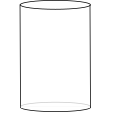b.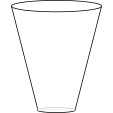c.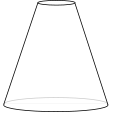d.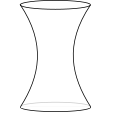e.### 5.

GCSE Higher

The diagram is of a container which is filled with water entering at a constant rate.Which of the following might be the graph of height of the water in the container plotted against time?

a.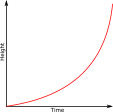b.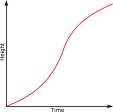c.d.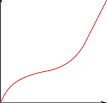e.### 6.

GCSE Higher

Match the equation with the letter of its graph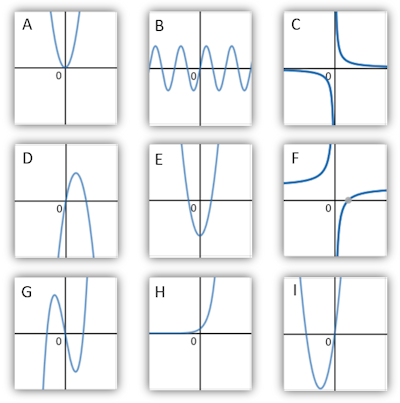EquationGraph
$$y=3-\frac{10}{x}$$
$$y=2^x$$
$$y=\sin x$$
$$y=x^2+7x$$
$$y=x^2-8$$
$$y=2-x$$

### 7.

GCSE Higher

The graph of y = f(x) is drawn accurately on the grid.

(a) Write down the coordinates of the turning point of the graph.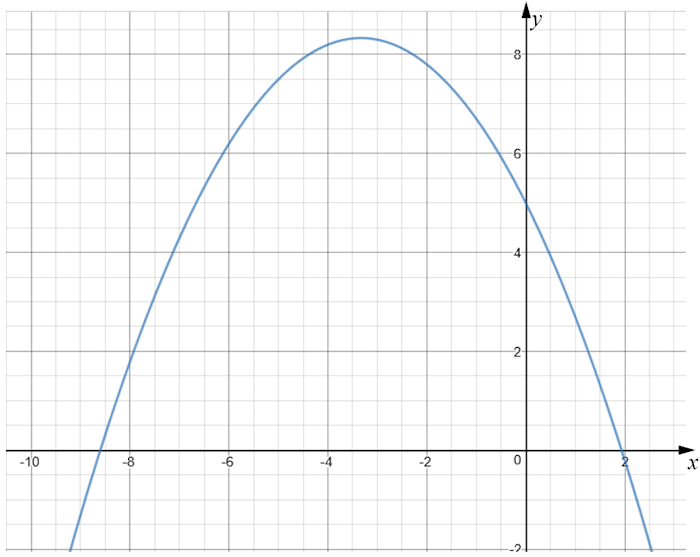(b) Write down estimates for the roots of f(x) = 0

(c) Use the graph to find an estimate for f(-5.5).

### 8.

GCSE Higher

(a) By completing the square, solve $$x^2+8x+13=0$$ giving your answer to three significant figures.

(b) From the completed square you found in part (a) find the minimum value of the curve $$y=x^2+8x+13$$.

### 9.

GCSE Higher

Suppose a rhombus ABCD is drawn on a coordinate plane with the point A situated at (4,7). The diagonal BD lies on the line $$y = 2x - 5$$

Find the equation the line that passes through A and C.

### 10.

GCSE Higher

The graph shows the temperature ($$T$$) of an unidentified flying object over a period of 10 seconds ($$t$$).Use the graph to work out an estimate of the rate of decrease of temperature at 7 seconds. You must show your working.

### 11.

GCSE Higher

The graph of the following equation is drawn and then reflected in the x-axis

$$y = 2x^2 - 3x + 2$$

(a) What is the equation of the reflected curve?

The original curve is reflected in the y-axis.

(b) What is the equation of this second reflected curve?

### 12.

GCSE Higher

(a) Find the interval for which $$x^2 - 9x + 18 \le 0$$

(b) The point (-4, -4) is the turning point of the graph of $$y = x^2 + ax + b$$, where a and b are integers. Find the values of a and b.

### 13.

GCSE Higher

(a) Write $$2x^2+8x+27$$ in the form $$a(x+b)^2+c$$ where $$a$$, $$b$$, and $$c$$ are integers, by 'completing the square'

(b) Hence, or otherwise, find the line of symmetry of the graph of $$y = 2x^2+8x+27$$

(c) Hence, or otherwise, find the turning point of the graph of $$y = 2x^2+8x+27$$

### 14.

IB Standard

A function is defined as $$f(x) = 2{(x - 3)^2} - 5$$ .

(a) Show that $$f(x) = 2{x^2} - 12x + 13$$.

(b) Write down the equation of the axis of symmetry of this graph.

(c) Find the coordinates of the vertex of the graph of $$f(x)$$.

(d) Write down the y-intercept.

(e) Make a sketch the graph of $$f(x)$$.

Let $$g(x) = {x^2}$$. The graph of $$f(x)$$ may be obtained from the graph of $$g(x)$$ by the two transformations:

• a stretch of scale factor $$s$$ in the y-direction;
• followed by a translation of $$\left( {\begin{array}{*{20}{c}} j\\ k \end{array}} \right)$$ .

(f) Find the values of $$j$$, $$k$$ and $$s$$.

### 15.

IB Studies

Consider a straight line graph L1, which intersects the x-axis at A(8, 0) and the y-axis at B (0, 4).

(a) Write down the coordinates of C, the midpoint of line segment AB.

(b) Calculate the gradient of the line L1.

The line L2 is parallel to L1 and passes through the point (5 , 9).

(c) Find the equation of L2. Give your answer in the form $$ay + bx + c = 0$$ where $$a, b \text{ and } c \in \mathbb{Z}$$.

### 16.

IB Standard

$$f$$ and $$g$$ are two functions such that $$g(x)=3f(x+2)+7$$.

The graph of $$f$$ is mapped to the graph of $$g$$ under the following transformations:

A vertical stretch by a factor of $$a$$ , followed by a translation $$\begin{pmatrix}b \\c \\ \end{pmatrix}$$

Find the values of

(a) $$a$$;

(b) $$b$$;

(c) $$c$$.

(d) Consider two other functions $$h$$ and $$j$$. Let $$h(x)=-j(2x)$$. The point A(8, 7) on the graph of $$j$$ is mapped to the point B on the graph of $$h$$. Find the coordinates of B.

### 17.

IB Standard

Let $$f(x) = {x^2}$$ and $$g(x) = 3{(x+2)^2}$$ .

The graph of $$g$$ can be obtained from the graph of $$f$$ using two transformations.

(a) Give a full description of each of the two transformations.

(b) The graph of $$g$$ is translated by the vector $$\begin{pmatrix}-4\\5\\ \end{pmatrix}$$ to give the graph of $$h$$.

The point $$( 2{\text{, }}-1)$$ on the graph of $$f$$ is translated to the point $$P$$ on the graph of $$h$$.

Find the coordinates of $$P$$.

### 18.

IB Standard

Let $$f$$ and $$g$$ be functions such that $$g(x) = 3f(x - 2) + 7$$ .

The graph of $$f$$ is mapped to the graph of $$g$$ under the following transformations: vertical stretch by a factor of $$k$$ , followed by a translation $$\left( \begin{array}{l} p\\ q \end{array} \right)$$ .

Write down the value of:

(a) $$k$$

(b) $$p$$

(c) $$q$$

(d) Let $$h(x) = - g(2x)$$ . The point A($$8$$, $$7$$) on the graph of $$g$$ is mapped to the point $${\rm{A}}'$$ on the graph of $$h$$ . Find $${\rm{A}}'$$

### 19.

IB Standard

Let $$f(x) = \frac{9x-3}{bx+9}$$ for $$x \neq -\frac9b, b \neq 0$$.

(a) The line $$x = 3$$ is a vertical asymptote to the graph of $$f$$. Find the value of b.

(b) Write down the equation of the horizontal asymptote to the graph of $$f$$.

(c) The line $$y = c$$ , where $$c\in \mathbb R$$ intersects the graph of $$\begin{vmatrix}f(x) \end{vmatrix}$$ at exactly one point. Find the possible values of $$c$$.

### 20.

IB Standard

Two functions are defined as follows: $$f(x) = 2\ln x$$ and $$g(x) = \ln \frac{x^2}{3}$$.

(a) Express $$g(x)$$ in the form $$f(x) - \ln a$$ , where $$a \in {{\mathbb{Z}}^ + }$$ .

(b) The graph of $$g(x)$$ is a transformation of the graph of $$f(x)$$ . Give a full geometric description of this transformation.

The exam-style questions appearing on this site are based on those set in previous examinations (or sample assessment papers for future examinations) by the major examination boards. The wording, diagrams and figures used in these questions have been changed from the originals so that students can have fresh, relevant problem solving practice even if they have previously worked through the related exam paper.

The solutions to the questions on this website are only available to those who have a Transum Subscription.

Exam-Style Questions Main Page

Search for exam-style questions containing a particular word or phrase:

To search the entire Transum website use the search box in the grey area below.

Kausar Begum Khan, Kenya

Friday, January 26, 2018

"This is a fabulous website! I cannot thank you enough."

Tuesday, November 6, 2018

"Transum is so resourceful. The resources are so child-friendly that my students love its usage.It has a wide range of topics and caters to most of my needs. 'Thankyou" will be too small a word to express my gratitude to the content developers of this site."

Ahmed Jaja, Ghana

Thursday, November 22, 2018

"It was very useful for me.I recommend it to every math student.Thank you."

Tuesday, February 5, 2019

"A very great site.Nice questions."

Yuli, Woodmansterne

Tuesday, April 2, 2019

"This website is amazing it helps me with my maths to get good marks in my upcoming test. Thanks."

Do you have any comments? It is always useful to receive feedback and helps make this free resource even more useful for those learning Mathematics anywhere in the world. Click here to enter your comments.For All: